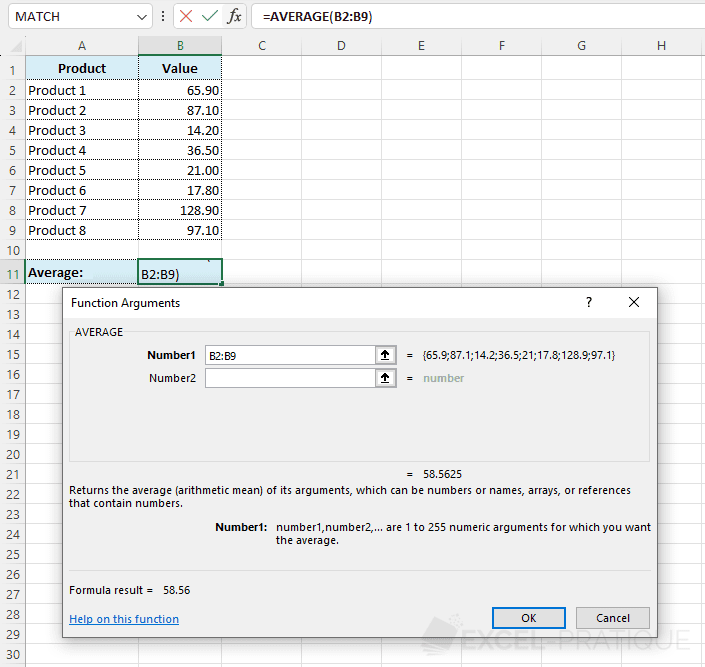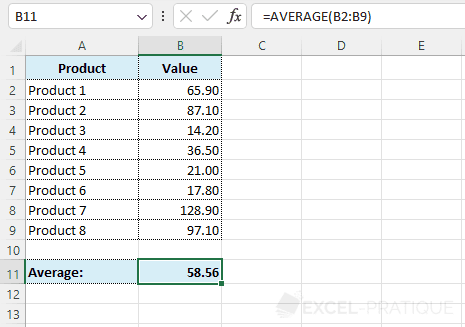# Excel Function: AVERAGE

The Excel function AVERAGE returns the average of a series of values.

Usage:

`=AVERAGE(range_of_values)`

or

`=AVERAGE(value_1, value_2, value_3, etc)`

## Example of use

Select the AVERAGE function and enter in Number1 the range of cells containing the values:Click OK.

The average value is then displayed: